# Two-Pentomino Balanced Rectangles

• Introduction
• Nomenclature
• Table
• Solutions
• Square Solutions
• ## Introduction

A pentomino is a figure made of five squares joined edge to edge. There are 12 such figures, not distinguishing reflections and rotations. They were first enumerated and studied by Solomon Golomb.

It has long been known that only four pentominoes can tile rectangles: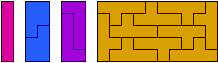For other rectangles that these pentominoes tile, see Mike Reid's Rectifiable Polyomino Page.

Rodoflo Kurchan's online magazine Puzzle Fun studied the problem of tiling some rectangle with two different pentominoes, in Issue 19, and revisited the problem in Issue 21. The August 2010 issue of Erich Friedman's Math Magic broadened this problem to use two polyominoes of any size, not necessarily the same.

Here I study the related problem of tiling some rectangle with two pentominoes, using the same number of copies of each.

For other sizes of polyominoes, see issue 26 of Puzzle Fun.

## Nomenclature

I use Solomon W. Golomb's original names for the pentominoes: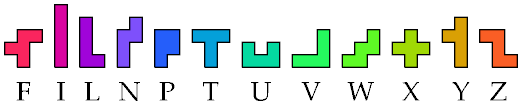## Table

This table shows the smallest total number of pentominoes known to be able to tile a rectangle in equal numbers.

FILNPTUVWXYZ
F * 28 4 × 8 × 12 4 × × 8 ×
I 28 * 4 24 4 56 ? 12 56 ? 8 60
L 4 4 * 4 4 24 4 4 4 ? 8 32
N × 24 4 * 4 24 4 4 × × 8 ×
P 8 4 4 4 * 12 4 6 8 40 4 12
T × 56 24 24 12 * 96 × 64 × 4 ×
U 12 ? 4 4 4 96 * 136 × × 12 ×
V 4 12 4 4 6 × 136 * 72 × 12 4
W × 56 4 × 8 64 × 72 * × 14 ×
X × ? ? × 40 × × × × * 20 ×
Y 8 8 8 8 4 4 12 12 14 20 * 8
Z × 60 32 × 12 × × 4 × × 8 *

## Solutions

So far as I know, these solutions have minimal area. They are not necessarily uniquely minimal.

### 4 Tiles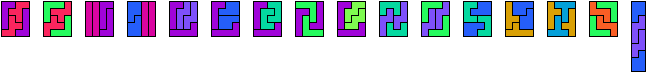### 6 Tiles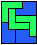### 8 Tiles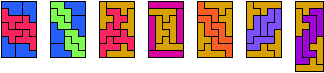### 12 Tiles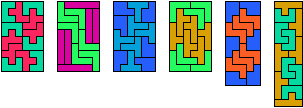### 14 Tiles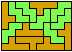### 20 Tiles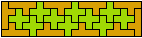### 24 Tiles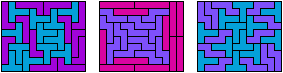### 28 Tiles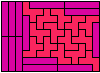### 32 Tiles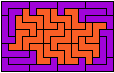### 40 Tiles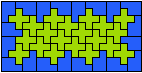### 56 Tiles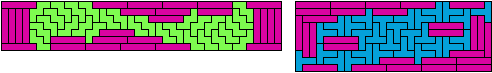### 60 Tiles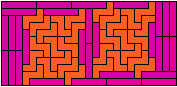### 64 Tiles### 72 Tiles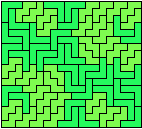### 96 Tiles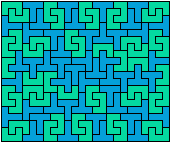### 136 Tiles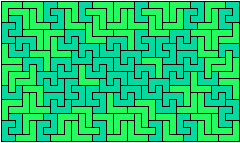## Square Solutions

### 20 Tiles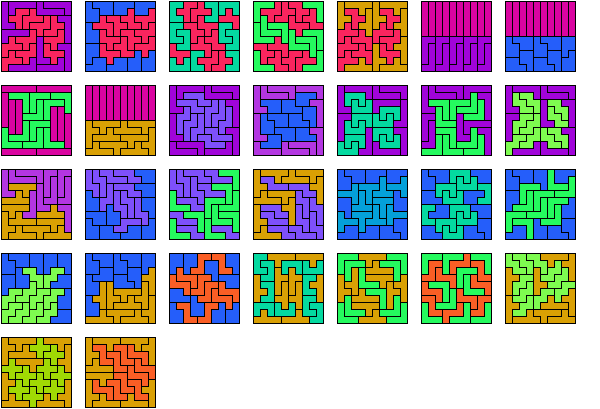### 80 Tiles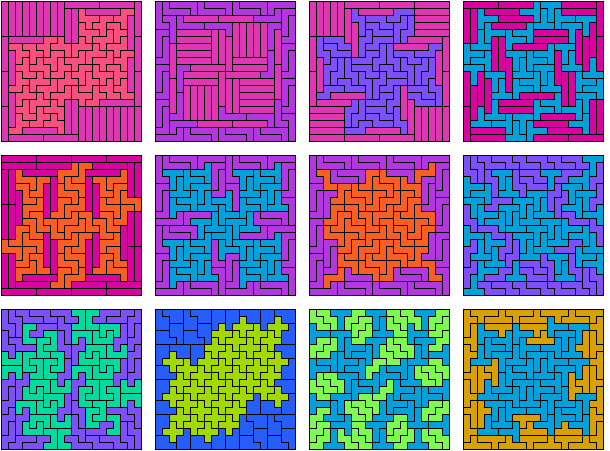### 180 Tiles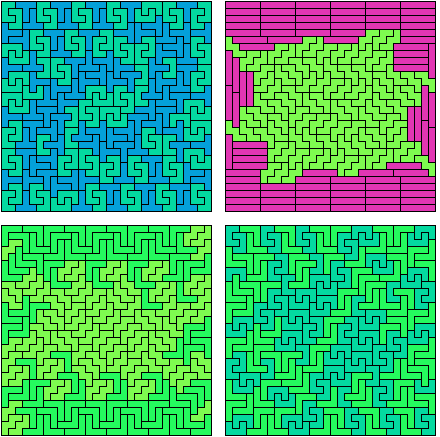Last revised 2013-04-08.

Back to Polyform Tiling < Polyform Curiosities
Col. George Sicherman [ HOME | MAIL ]